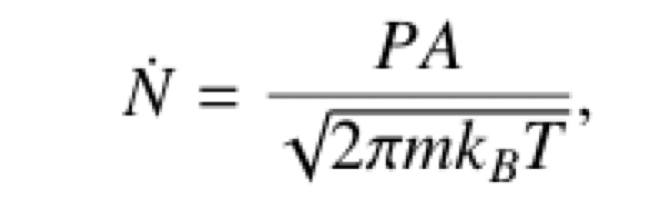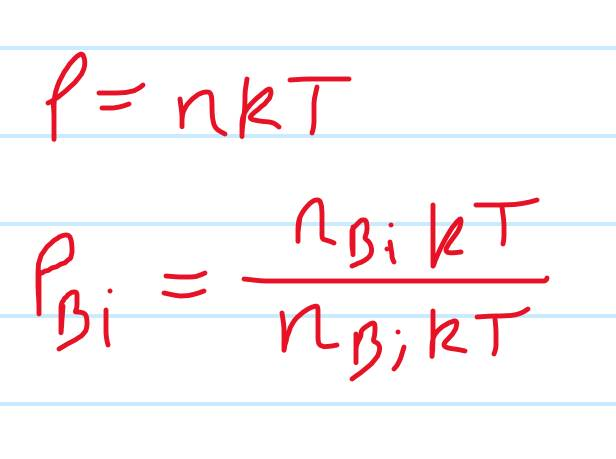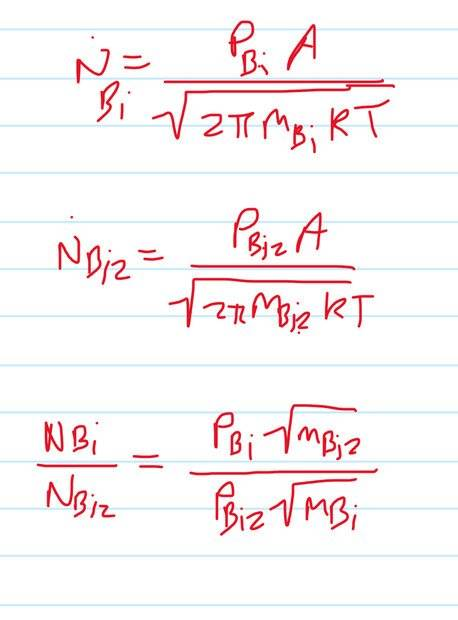# Ratio of partial pressures of gas

so_gr_lo
Homework Statement:
Bismuth is heated to 1100 K in an oven and gives rise to a beam of vapour molecules effusions from an aperture of area 10^-6 m^2. The beam contains Bi and Bi2 molecules in the ratio 1.41:1. Calculate the ratio of the partial pressures of Bi to Bi2 molecules

I believe the total pressure is the sum of the partial pressures but I’m not sure what to do with that, so I tried just calculating the ratio of the pressures but don’t know where to go from there
Relevant Equations:
P=nKT where n is the number density per unit volume

and rate of effusion formula given below
Rate of effusionHomework Helper
Gold Member
2022 Award
Just guessing here, but try writing your rate of effusion equation for each species separately. Each has its own pressure, mass and effusion rate, but they have the same temperature and area.

so_gr_lo
So this is what I get. since the rate of effusion is proportional to the number of molecules I guess you just plug the given number ratio and masses in and solve for the pressure ratio.